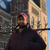# New to Qlik Sense

If you’re new to Qlik Sense, start with this Discussion Board and get up-to-speed quickly.

Announcements
Customer & Partners, DEC. 9, 11 AM ET: Qlik Product & Strategy Roadmap Session: Data Analytics REGISTER NOW
cancel
Showing results for
Did you mean:Contributor III

## Measure x Dimension SUM() problem

Hello i have a calculated column that looks like this:

(( Column1 *Column2 ) /1000) / Column3 AS CalculatedColumn

This column seems to be calculated right. As example it has this values:

((2385*25)/1000)/24,47 = 2,43 wich is right and when i add it as Dimension to table it shows this right value

but when i try to use Sum() function like Sum(CalculatedColumn) the output is recalculated as 4908,7 wich is not right.

So the column has right values as dimension but not as measure with Sum() function

I was thinking that it is maybe some Formatting problem ? Or how to solve this ?